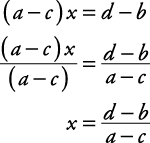## Equations

An equation is a mathematical sentence, a relationship between numbers and/or symbols that says two expressions are of equal value.

#### Axioms of equality

For all real numbers a, b, and c, the following are some basic rules for using the equal sign.

• Reflexive axiom: a = a.

Therefore, 4 = 4.

• Symmetric axiom: If a = b, then b = a.

Therefore, if 2 + 3 = 5, then 5 = 2 + 3.

• Transitive axiom: If a = b and b = c, then a = c.

Therefore, if 1 + 3 = 4 and 4 = 2 + 2, then 1 + 3 = 2 + 2.

• Additive axiom of equality: If a = b and c = d, then a + c = b + d.

Therefore, if 1 + 1 = 2 and 3 + 3 = 6, then 1 + 1 + 3 + 3 = 2 + 6.

• Multiplicative axiom of equality: If a = b and c = d, then ac = bd.

Therefore, if 1 =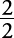and 4 =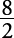, then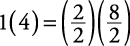.

#### Solving equations

Remember that an equation is like a balance scale with the equal sign (=) being the fulcrum, or center. Thus, if you do the same thing to each side of the equation (say, add 5 to each side), the equation will still be balanced.

##### Example 1

Solve for x.

x – 5 = 23

To solve the equation x – 5 = 23, you must get x by itself on one side; therefore, add 5 to each side of the equation.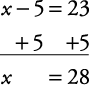In the same manner, you may subtract, multiply, or divide both sides of an equation by the same (nonzero) number, and the solution will not change. Sometimes you may have to use more than one step to solve for an unknown.

##### Example 2

Solve for x.

3 x + 4 = 19

Subtract 4 from each side of the equation to get the 3 x by itself on one side.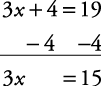Then divide each side of the equation by 3 to get x.Remember that solving an equation is using opposite operations until the letter is on a side by itself (for addition, subtract; for multiplication, divide; and so forth).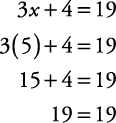##### Example 3

Solve for x.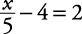Add 4 to each side of the equation.Multiply each side of the equation by 5 to get x.##### Example 4

Solve for x.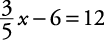Add 6 to each side of the equation.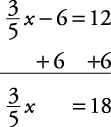Multiply each side of the equation by(same as dividing by).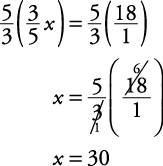##### Example 5

Solve for x.

5 x = 2 x – 6

Subtract 2 x from each side of the equation.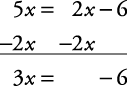Divide each side of the equation by 3.##### Example 6

Solve for x.

6 x + 3 = 4 x + 5

Subtract 4 x from each side of the equation.Subtract 3 from each side of the equation.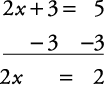Divide each side of the equation by 2.#### Literal equations

Literal equations have no numbers, only symbols (letters).

##### Example 7

Solve for q.

qpx = y

First add x to each side of the equation.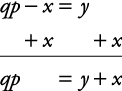Then divide each side of the equation by p.Operations opposite to those in the original equation were used to isolate q. (To remove the –x, an x was added to each side of the equation. Because the problem has q times p, each side of the equation was divided by p.

##### Example 8

Solve for y.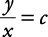Multiply each side of the equation by x to get y alone.##### Example 9

Solve for x.

ax + b = cx + d

Subtract cx from each side of the equation.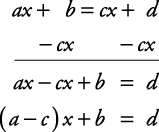ax – cx = ( a – c) x by the distributive property.

Subtract b from each side of the equation.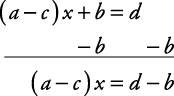Divide each side of the equation by ( a – c).